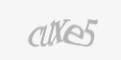Blog

360dissertations understands how tough Masters level projects can be. The standard of a post graduate course is higher than a bachelor’s degree.

Research often involves circumstances where the assumption of normality is violated. This is when a statistical test such as logistic regression involving non-normal variables come handy. Logistic regression, known as the logit model, is an analytical method to examine/predict the direct relationship within an independent variable and dependent variables. It is used to model binary result variables. Today, this statistical technique is considered to be one of the most commonly used methods to predict a real-valued result.

Logistic regression is a particular case of the generalized linear model where the dependent variable or response variable is either a 0 or 1. It returns the target variables as probability values. However, we can transform and obtain the benefits using threshold value. It should be noted that logistic regression considers only the probability of the dependent variable and uses Generalized Linear Model (GLM).

To successfully perform logistic regression test a few assumptions need to be considered. This includes:

The dependent or response variable should be binomially distributed.

There should be a linear relationship between the independent variables and the logit or link function.

The response variable must be mutually exclusive.

Typically, logistic regression can be calculated using equations like,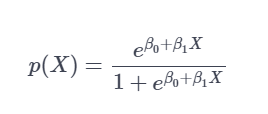The above equation can be transformed as: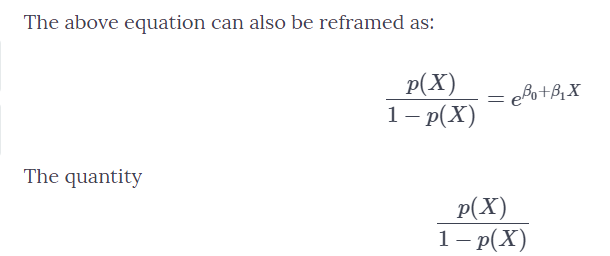Taking log on both sides the final equation can be written as :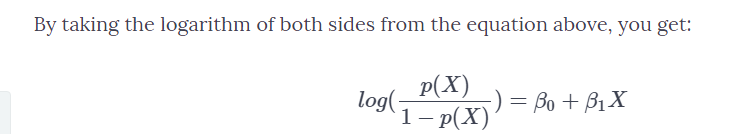Although statistical tools such as python, sklearn, statsmodel, etc. can be used to perform this test, R language is one tool that is the right-fit for performing logistic regression. This is because R is open-source software, which has a wide variety of libraries and packages available to perform this statistical test with ease. However, prior to conducting the test, it is a must to clean the data, failing to which the output obtained may be inaccurate.

Steps involved in performing logistic regression in R language

Consider an example of binary logistic regression which is analysed using ISLR package.

Step 1: This step involves installing and loading the ISLR package. To do so, use the data sets present in the package and then apply the model on real data set.

Note: names() is used to check the names & data in the data frame

head() is a glimpse of the first few rows.

summary() is a function used for getting all the major insights of the data.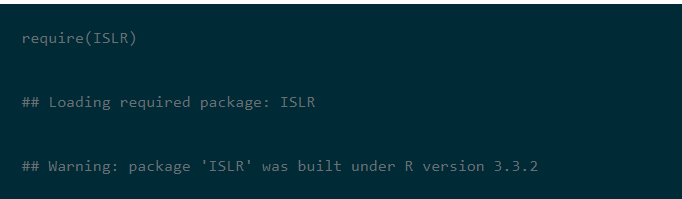The density box gives an insight into the separation of Up and Down. It creates an overlapping picture in direction value. Also, GLM () function is used to implement generalized linear models.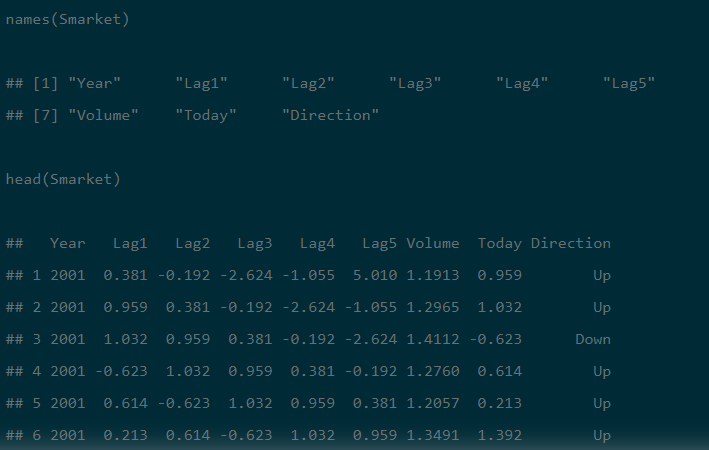Step 2:  The library  “CARET ” should be installed and then used to list down all the variables. This is followed by treating the outliers, missing values and create new variables using feature engineering technique and then train the sample.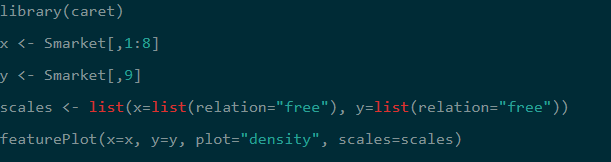Step 3: Here the summary() has to be returned to all the estimates, standard errors, z-score, and p-values for every coefficient. In the below example, it is observed that none of the coefficients are significant. This step gives us the null deviance and the residual deviance, with a minimal difference of 2 & 6 degrees of freedom.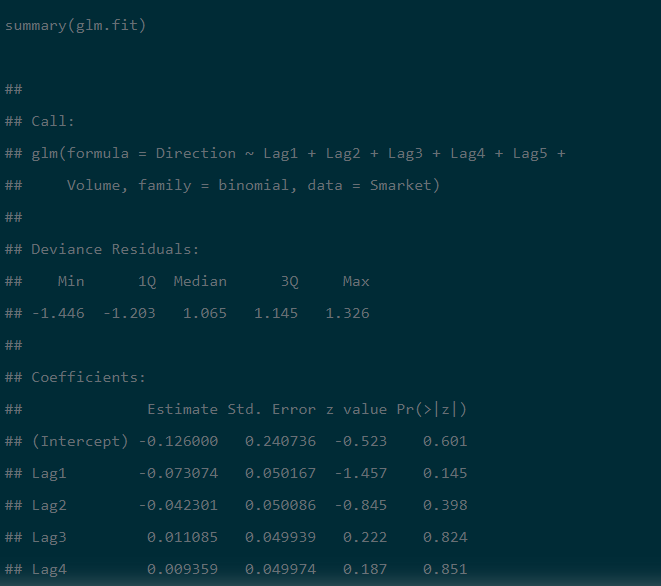Step 4: In the fourth step, training and test the samples. To make the process easier, segregate the sample into two trains and then test the sample.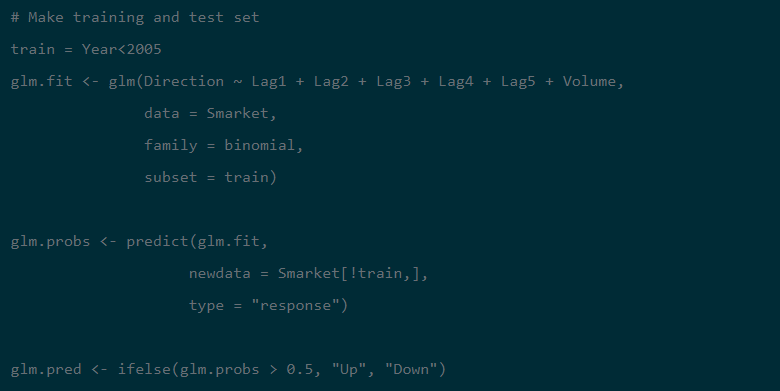STEP 5: Use the function Predict2 <- predict (Smarket, type=’response’) to predict the pending data.

STEP 6: Check the predictability and reliability of the model using ROC Curve

Note: ROC is an area under the curve which gives us the accuracy of the model. It is also known as the index of accuracy which represents the performance of the model.

To interpret, the greater the area under the curve, the better is the model.

Graphically it can be represented using two axis. Where one is true positive rate and another represents false positive rate. If the value approaches to 1 then better the model .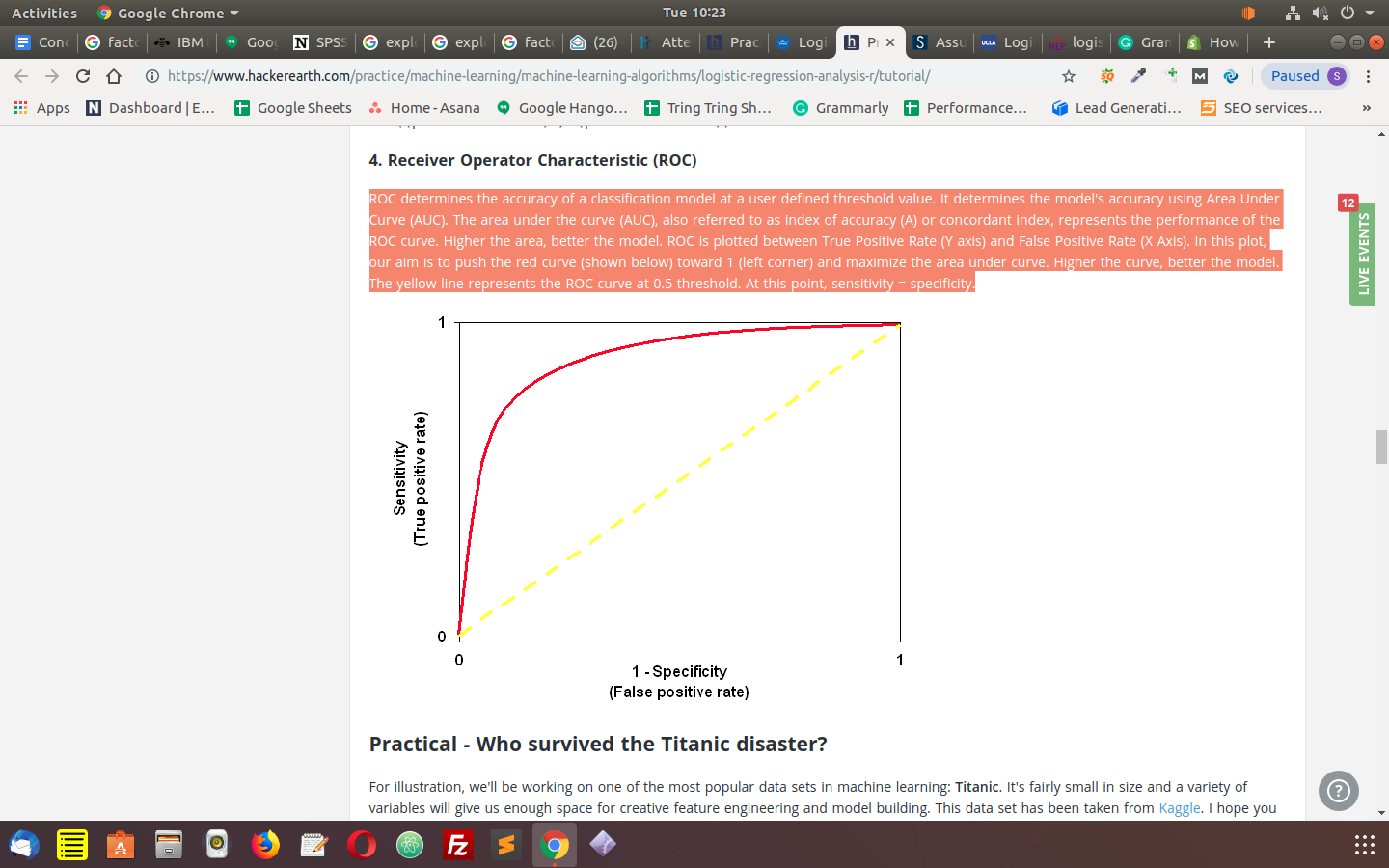The commands used to check the predictability are:

library(ROCR)

ROCRpred1 <- prediction(predict1, train\$Recommend)

ROCRperf1 <- performance(ROCRpred1, 'tr','fr')

plot(ROCRperf1, colorize = TRUE )

Now, plot glm function using ggplot2 library

library(ggplot2)

ggplot(train, aes(x=Rating, y=Recommend)) + geom_point() +

stat_smooth1(method="glm", family="binomial", se=FALSE)

GLM () model does not assume that there is any linear relationship between independent or dependent variables. Nevertheless, it does assume a linear relationship between link function and independent variables in the logistic model.

In the above example, a 59% classification rate is achieved. This indicates that a smaller model perform betters.